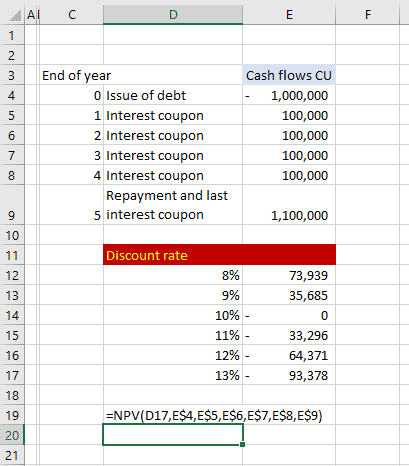Discount rate

As part of IFRS 13 Fair value measurement the measurement of fair value using the Discounted cash flow model has increased. One of the most influential inputs to a discounted cash flow model is the discount rate. A discount rate is used in different formats in different discounted models. How is this substantiated so your model is being taken seriously?

This will illustrate a way to build the equity discount rate used in WACC, DCF and/or Excess Earnings calculations. It will be build up as a specific discount rate for a specific company and always needs to be validated by comparison against recent transactions or so to provide a profound basis for calculating a value.

The discount rate is estimated as follows:

 Discount rate element Risk rate Notes Risk-free rate of return, such as a Government’s bond yield 3.00% Current US Treasury bond yield is used. Premium for equity investment 6.10% Risk-premium for listed company shares investment. Risk-premium for small company size 9.85% Risk premium for investing in a small company. Industry specific risk-premium 1.02% Risk depending on the industry, for example Management Consultancy Services Company-specific risk premium 2.50% Specific subject business risk premium Equity discount rate 22.47% SUM of above Net cash flow growth 3.52% Long-term growth rate in subject business Net Cash Flow (NCF). Capitalisation rate 18.95% Difference between the Equity Discount Rate and NCF Growth Rate above.

Discount Rate Sensitivity

When it comes to discounted cash flow analysis, your choice of discount rate can dramatically change your valuation. Consider the following chart showing the sensitivity of net present value to changes in the discount rate:As shown in the analysis above, the net present value for the given cash flows at a discount rate of 10% is equal to CU 0. This means that with an initial investment of exactly \$1,000,000, this series of cash flows will yield exactly 10% (the internal rate of return, IRR and the coupon rate of the debt (1,000,000 x 10% is 100,000). As the required discount rates moves higher than 10%, the investment becomes less valuable.

This happens because the higher the discount rate, the lower the initial investment needs to be in order to achieve the target yield. As you can see in the chart above, the selection of the discount rate can have a big impact on the discounted cash flow valuation.Discount rate

Discount rate Discount rate Discount rate Discount rate Discount rate Discount rate Discount rate Discount rate